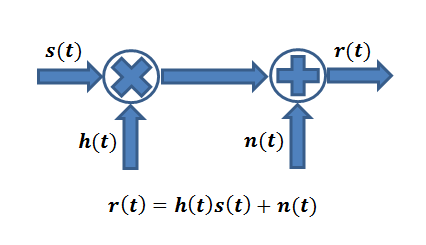# Wireless Channel Modeling: Back to FundamentalsSimplified Wireless Communication Channel Model

When a wireless signal travels from a transmitter (Tx) to a receiver (Rx) it undergoes some changes. In simple terms the signal s(t) is scaled by a factor h(t) and noise n(t) is added at the receiver. Let’s take this discussion forward with a simple example. Suppose the Tx transmits one of two possible symbols, +1 or -1. In technical lingo this is called Binary Phase Shift Keying (BPSK). If the channel scaling factor is 0.1 we will either get a +0.1 or -0.1 at the Rx to which AWGN noise is added. The noise is random in nature (having a Gaussian distribution) but for simplicity we assume that it can have one of two values, +0.01 or -0.01.

So if we transmitted a +1 from the Tx we can have one of two possible values at the Rx, 0.11 and 0.09. If the Rx is a simple sign detector we would be able to detect that +1 was transmitted. But what if the noise is higher, such that it can have values of +0.2 or -0.2? So after scaling by the channel and noise addition we could either get a +0.3 or -0.1 (0.1 ± 0.2). Clearly we now have a problem as there is a probability of sign flipping and sign detector may make a mistake. In simple terms scaling itself is not a problem but when noise is added to it the received signal gets corrupted and the sign detector can make a mistake.

But things are usually not so simple in a wireless environment. The channel sometimes creates multiple copies of the signal which overlap when they arrive at the Rx. Lets assume, as in our previous example that the scaling factor for both the copies of the signal is 0.1. So we receive 0.1+0.1=0.2 at the Rx to which noise is added. Now if the noise variable is no smaller than -0.2 the sign detector would still work and we will be able to recover the signal. Essentially the Signal to Noise Ratio (SNR) at the Rx increases when the two signal copies coherently add up.

But what if the channel does not only scale the signal but also flips the sign of one of the signal copies. The two signal copies cancel each other out and the signal is wiped out. This phenomenon is sometimes referred to as multipath fading. Typically the two signal copies do not completely cancel each other but the residual signal is so weak that it goes below the noise floor and is difficult to detect. There are numerous ways to improve the Signal to Noise Ratio; one of them is to use multiple antennas at the Rx to coherently add the signal copies when they arrive at the individual Rx antenna elements.

In practical wireless communication systems it is not uncommon to have six or more copies of the same signal arriving at the Rx. Also, channel scaling factor and noise typically are time varying, having a Rayleigh distribution and a Gaussian distribution respectively. Channel scaling is typically overcome by what is a called an equalizer and noise is removed by a filter. Lastly I would like to add that channel does not always simply scale but the effect is a bit more complicated and for this you need to understand the concept of convolution (please see this post).